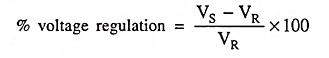## Performance of Short and Medium Transmission Lines Interview Questions and Answers:

1. What are the factors which govern the performance of a transmission line ?

Ans. The factors governing the performance of a transmission line are series resistance R and inductance L, shunt capacitance C and conductance G. However, the effect of conductance is negligible.

2. How do you classify transmission lines ?

Ans. Transmission lines are classified depending upon the manner in which capacitance is taken into account.

3. What are the causes of voltage drop and line loss in a transmission line ?

Ans. The voltage drop in a transmission line is due to its resistance and inductance whereas the line loss is due to its resistance.

4. What is voltage regulation of line ?

Ans. Regulation: When the load is supplied, there is a voltage drop in the line due to resistance and inductance of the line and, therefore, receiving-end voltage VR is usually less than sending-end voltage VS. The voltage drop i.e., difference of sending-end voltage and receiving-end voltage expressed as a percentage of receiving-end voltage is called the regulation.

When the load is thrown off i.e., when the line is supplying no load, the receiving-end voltage becomes equal to sending-end voltage and therefore, regulation can be defined as below :

Regulation is defined as the change in voltage at the receiving (or load) end when the full load is thrown off, the sending-end (or supply) voltage and supply frequency remaining unchanged. It is usually expressed as a percentage of receiving-end voltage.

Mathematically percentage voltage regulation of a transmission line is given by the expressionwhere VS is the voltage at sending or supply end and VR is the voltage at the receiving or load end. It is to be noted here that VS and VR are the magnitudes of voltages.

The lower the voltage regulation, better it is, because low voltage regulation means little variation in receiving-end voltage due to variation in load current.

Knowledge of voltage regulation helps in maintaining the voltage at the load terminals within prescribed limits by employing suitable voltage control equipment.

5. Explain the effect of load power factor on the transmission efficiency.

Ans. To deliver given power at a given voltage at consumer end, line current is inversely proportional to the power factor of the load. The current, hence, the power loss in the line goes on increasing as the power factor of the load goes on decreasing. Thus efficiency of the transmission line decreases with the fall in power factor and vice versa.

6. What do you understand by Ferranti effect ?

Ans. The phenomenon of rise in voltage at the receiving end of the open-circuited or lightly loaded line is called the Ferranti effect.

7. How does rise in voltage at the receiving end of an open-circuited line depend upon the length of the line and system operating voltage ?

Ans. Rise in voltage at the receiving end of an open-circuited line is proportional to the square of the length of the line and varies directly in proportion to the system operating voltage.

8. How do the terms ‘impedance drop’, ‘voltage drop’ and ‘voltage regulation’ , in connection with transmission lines differ ?

Ans. The impedance drop in a line is the voltage drop in the line impedance and is equal to the phase difference between the sending-ending voltage and the receiving-end voltage.

The voltage drop in a line is the arithmetical difference between the sending-end and receiving-end voltages.

The voltage regulation is defined as the change in voltage at the receiving-end when full-load is thrown off, the sending-end voltage being held constant.

9. Why in medium and long transmission lines, regulation is greater than voltage drop ?

Ans. The regulation is greater than voltage drop in a medium/long transmission line due to rise in voltage owing to Ferranti effect.

10. What are the units of generalized constants of a transmission line ?

Ans. Generalized constants A and D are dimensionless whereas the units of generalized constants B and C are ohms and siemens (mho) respectively.

Scroll to Top